Courses

# Test: Single Correct MCQs: Heat & Thermodynamics | JEE Advanced

## 9 Questions MCQ Test Physics For JEE | Test: Single Correct MCQs: Heat & Thermodynamics | JEE Advanced

Description
This mock test of Test: Single Correct MCQs: Heat & Thermodynamics | JEE Advanced for JEE helps you for every JEE entrance exam. This contains 9 Multiple Choice Questions for JEE Test: Single Correct MCQs: Heat & Thermodynamics | JEE Advanced (mcq) to study with solutions a complete question bank. The solved questions answers in this Test: Single Correct MCQs: Heat & Thermodynamics | JEE Advanced quiz give you a good mix of easy questions and tough questions. JEE students definitely take this Test: Single Correct MCQs: Heat & Thermodynamics | JEE Advanced exercise for a better result in the exam. You can find other Test: Single Correct MCQs: Heat & Thermodynamics | JEE Advanced extra questions, long questions & short questions for JEE on EduRev as well by searching above.
QUESTION: 1

### A fixed thermally conducting cylinder has a radius R and height L0. The cylinder is open at its bottom and has a small hole at its top. A piston of mass M is held at a distance L from the top surface, as shown in the figure. The atmospheric pressure is P0.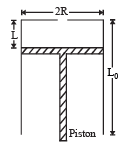Q.1. The piston is now pulled out slowly and held at a distance 2L from the top. The pressure in the cylinder between its top and the piston will then be

Solution:

NOTE : When the piston is pulled out slowly, the pressure drop produced inside the cylinder is almost instantaneously neutralised by the air entering from outside into the cylinder (through the small hole at the top).
Therefore, the pressure inside the cylinder is P0 throughout the slow pulling process.

QUESTION: 2

### A fixed thermally conducting cylinder has a radius R and height L0. The cylinder is open at its bottom and has a small hole at its top. A piston of mass M is held at a distance L from the top surface, as shown in the figure. The atmospheric pressure is P0.Q.2. While the piston is at a distance 2L from the top, the hole at the top is sealed. The piston is then released, to a position where it can stay in equilibrium. In this condition, the distance of the piston from the top is

Solution:

KEY CONCEPT : The condition for equilibrium of the piston is

Mg = (P0 – p) πR2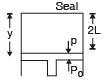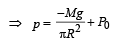NOTE : Since the cylinder is thermally conducting, the temperature  remains the same.
Therefore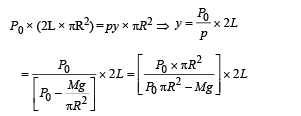QUESTION: 3

### A fixed thermally conducting cylinder has a radius R and height L0. The cylinder is open at its bottom and has a small hole at its top. A piston of mass M is held at a distance L from the top surface, as shown in the figure. The atmospheric pressure is P0.Q.3. The piston is taken completely out of the cylinder. The hole at the top is sealed. A water tank is brought below the cylinder and put in a position so that the water surface in the tank is at the same level as the top of the cylinder as shown in the figure. The density of the water is r. In equilibrium, the height H of the water column in the cylinder satisfies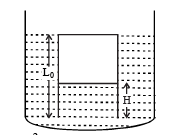Solution:

At equilibrium, p = P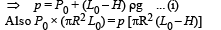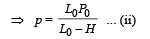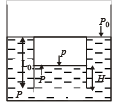From (i) and (ii)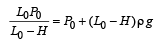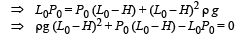QUESTION: 4

A small spherical monoatomic ideal gas bubble (γ = 5/3) is trapped inside a liquid of density ρ (see figure). Assume that the bubble does not exchange any heat with the liquid. The bubble contains n moles of gas. The temperature of the gas when the bubble is at the bottom is T0, the height of the liquid is H and the atmospheric pressure is P0 (Neglect surface tension).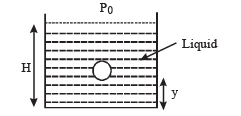Q.4. As the bubble moves upwards, besides the buoyancy force the following forces are acting on it

Solution:

The forces acting besides buoyancy force are (a) Force of gravity (vertically downwards) (b) Viscous force (vertically downwards)

QUESTION: 5

A small spherical monoatomic ideal gas bubble (γ = 5/3) is trapped inside a liquid of density ρ (see figure). Assume that the bubble does not exchange any heat with the liquid. The bubble contains n moles of gas. The temperature of the gas when the bubble is at the bottom is T0, the height of the liquid is H and the atmospheric pressure is P0 (Neglect surface tension).Q.5. When the gas bubble is at a height y from the bottom, its temperature is –

Solution:

t is given that the bubble does not exchange any heat with the liquid. This means that while the bubble moves up and expand, the process is adiabatic.
For adiabatic expansion the pressure -temperature relationship is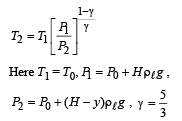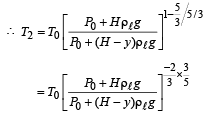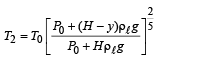QUESTION: 6

A small spherical monoatomic ideal gas bubble (γ = 5/3) is trapped inside a liquid of density ρ (see figure). Assume that the bubble does not exchange any heat with the liquid. The bubble contains n moles of gas. The temperature of the gas when the bubble is at the bottom is T0, the height of the liquid is H and the atmospheric pressure is P0 (Neglect surface tension).Q.6. The buoyancy force acting on the gas bubble is (Assume R is the universal gas constant)

Solution:

Buoyancy force = weight of fluid displaced = (mass of fluid displaced) g

= Vρlg    ...(ii)

where V = Volume of fluid displaced                 = Volume of the bubble.
Now, PV = nRT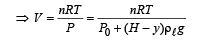Where P is pressure of the bubble at an arbitrary location distant y from the bottom.
Substituting the value of tempertaure from equtaion (i) we get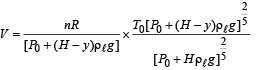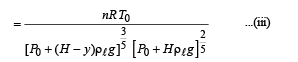From (ii) and (iii)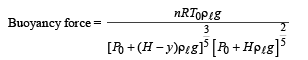QUESTION: 7

In the figure, a container is shown to have a movable (without friction) piston on top. The container and the piston are all made of perfectly insulated material allowing no heat transfer between outside and inside the container. The container is divided into two compartments by a rigid partition made of a thermally conducting material that allows slow transfer of heat. The lower compartment of the container is filled with 2 moles of an ideal monatomic gas at 700 K and the upper compartment is filled with 2 moles of an ideal diatomic gas at 400 K. The heat capacities per mole of an ideal monatomic gas are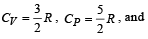those for an ideal diatomic gas are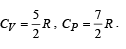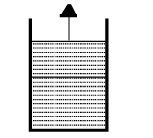Q.7. Consider the partition to be rigidly fixed so that it does not move. When equilibrium is achieved, the final temperature of the gases will be

Solution:

Heat lost by monatomic gas at constant volume = Heat gained by diatomic gas at constant pressure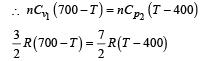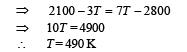QUESTION: 8

In the figure, a container is shown to have a movable (without friction) piston on top. The container and the piston are all made of perfectly insulated material allowing no heat transfer between outside and inside the container. The container is divided into two compartments by a rigid partition made of a thermally conducting material that allows slow transfer of heat. The lower compartment of the container is filled with 2 moles of an ideal monatomic gas at 700 K and the upper compartment is filled with 2 moles of an ideal diatomic gas at 400 K. The heat capacities per mole of an ideal monatomic gas arethose for an ideal diatomic gas areQ.8.  Now consider the partition to be free to move without friction so that the pressure of gases in both compartments is the same. The total work done by the gases till the time they achieve equilibrium will be

Solution:

In this case both the gases are at constant pressure.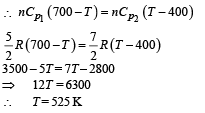Applying first law of thermodynamics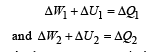As the gas two system is thermally insulated, therefore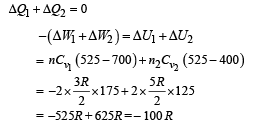Therefore, total work done = –100 R

QUESTION: 9

Statement-1 : The total translational kinetic energy of all the molecules of a given mass of an ideal gas is 1.5 times the product of its pressure and its volume.  because

Statement-2 : The molecules of a gas collide with each other and the velocities of the molecules change due to the collision.

Solution:

Statement 1 : The total kinetic energy of n moles of gas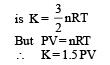Statement one is true.
Statement 2 :  The molecules of a gas collide with each other and the velocities of the molecules change due to collision.
But statement 2 is not a correct explanation of statement 1.